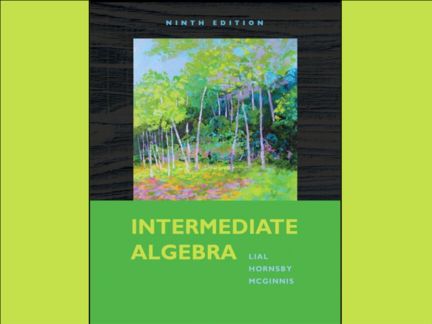# Rational Expressions and Functions; Multiplying and DividingContributed by:OBJECTIVES:
1. Define rational expressions. 2. Define rational functions and describe their domains. 3. Write rational expressions in lowest terms. 4. Multiply rational expressions. 5. Find reciprocals for rational expressions. 6. Divide rational expressions.
Sec 8.1 - 1
2. Chapter 8
Rational Expressions and
Functions
Sec 8.1 - 2
3. 8.1
Rational Expressions and
Functions;
Multiplying and Dividing
Sec 8.1 - 3
4. 8.1 Rational Expressions and Functions;
Multiplying and Dividing
Objectives
1. Define rational expressions.
2. Define rational functions and describe
their domains.
3. Write rational expressions in lowest terms.
4. Multiply rational expressions.
5. Find reciprocals for rational expressions.
6. Divide rational expressions.
5. 8.1 Rational Expressions and Functions;
Multiplying and Dividing
Defining Rational Expressions
In Section 1.1, we defined rational numbers to be the quotient
of two integers, a / b with b not equal to 0.
A rational expression (algebraic fraction) is the quotient of two
polynomials, also with the denominator not 0. Rational
expressions are the elements of the set
6. 8.1 Rational Expressions and Functions;
Multiplying and Dividing
Define Rational Functions and Describe Their Domain
A rational function has the form
The domain of a rational function includes all the real numbers
except those that make Q(x), the denominator, equal to 0.
7. 8.1 Rational Expressions and Functions;
Multiplying and Dividing
Define Rational Functions and Describe Their Domain
The graph of the function
f(x) is shown at the right.
The domain of this function
is all real numbers except
2
x = 3 where f(x) is not
3
8. 8.1 Rational Expressions and Functions;
Multiplying and Dividing
Finding Numbers Not in the Domain of a Rational Function
To locate the values not in the domain of a rational function,
we need only determine which real numbers make the
denominator 0.
3
9. 8.1 Rational Expressions and Functions;
Multiplying and Dividing
Writing Rational Expressions in Lowest Terms
3
10. 8.1 Rational Expressions and Functions;
Multiplying and Dividing
Writing Rational Expressions in Lowest Terms
3
11. 8.1 Rational Expressions and Functions;
Multiplying and Dividing
When the Numerator and Denominator are Opposites
3
12. 8.1 Rational Expressions and Functions;
Multiplying and Dividing
Multiplying Rational Expressions
3
13. 8.1 Rational Expressions and Functions;
Multiplying and Dividing
Multiplying Rational Expressions
3
14. 8.1 Rational Expressions and Functions;
Multiplying and Dividing
Finding the Reciprocal of a Rational Expression
To find the
3
reciprocal, simply
interchange the
numerator and
denominator.